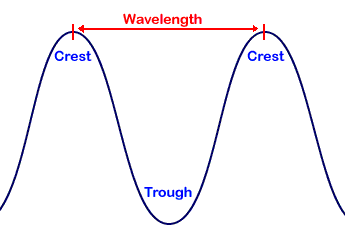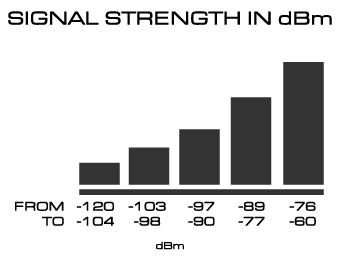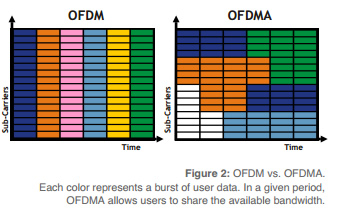# Guide to Understanding TerminologyThis common language guide will help familiarise you with the units, acronyms, abbreviations and technical terms used in telecommunications.

WavelengthA radio wave is not that different to an ocean's wave - in the sense of its shape. The distance from the top of the wave (crest), to the top of the following wave is commonly referred to as its wavelength.

MHz: (Megahertz)

The wavelength of a radio frequency is measured in megahertz.

A Hertz refers to the number of waves per second eg. 100Hz = “100 cycles per second”. So the Telstra Next-G network sends out a radio wave into the atmosphere at 850,000,000 cycles per second.

Gain

One of the most common misconceptions about antenna gain is that a higher gain amplifies the signal.

Gain actually describes how the antenna causes the signal to focus more in some directions than in others.

So a higher gain antenna actually doesn't increase the power of the signal, it just focuses it more in one direction to create a denser signal that travels further.

Conversely, a low gain antenna is less focused, and as such the signal travels higher and lower, but less distance.

dB (Decibel)

dB is a logarithmic comparison between two quantities - this description sounds a little abstract, so let's give it some context.

You might already be familiar with dB in measuring sound levels, where the comparison is between the threshold of human hearing (i.e. audible silence), and something we're trying to measure.

For example, a conversation is 60dB, a lawn mower is 90dB, and a jet engine is 120dB. You might be thinking, how can a jet engine be only twice as many dB as a conversation? This is because dB is a base-10 logarithmic scale, meaning a 10dB increase = 100x as loud, 20dB increase = 1000x as loud, and so on.

When it comes to antennas, we're no longer comparing things to the threshold of human hearing, instead we often compare against a reference antenna, or the level of signal we have at a particular point.

Remember - several different measures of 'dB' exist and all differ depending on different applications.

dBi (Decibel-isotropic)

This is the standard measure of antenna gain. It is a comparison between the gain of a real antenna to that of a theoretical 'isotropic' antenna, which distributes signal evenly in a perfectly spherical pattern (i.e. it is the zero point - 0dBi).dBm (Decibel-milliwatt)

This is the most common measure of signal strength. It refers to an absolute measure of power in terms of milliwatts (mW) and is expressed with 0dBm = 1mW.

Because most wireless broadband transmissions take place at extremely low wattage (far less than 1mW) the value is always negative.

Signal strength will often range between -60dBm and -120dBm, with -60dBm being a very strong signal, and -120dBm being almost no signal whatsoever.

dBq (Decibel Quarter-Wave)

This is a rarely used measure of antenna gain, with its reference point being the gain over a quarter-wave monopole. Effectively 0.85dBi = 1dBq.

Received Signal Strength Indication. RSSI is the technical abbreviation for how much raw signal (in dBm) is being received by your modem, and is often displayed graphically as a number of bars of signal.

This is one of our most important measures and luckily is quite easy to understand.

The downside of this measure is that it is a measure of total signal, including noise.

Ec/Io

This is a ratio of Signal to Interference.

Similar to a Signal to Noise (SNR) ratio, this is simply a measure of signal quality as opposed to raw signal strength.

Ec/Io value will often vary between -10 (best quality) and -40 (worst quality). If you want a more in depth explanation, I recommend, What is Ec/Io (and Eb/No)?

RSCP

Received Signal Code Power. This measure is specific to networks that use code based modulation techniques, it is calculated by adding the two previous mentioned measures.

This is used to give an indication of overall signal strength and quality in dBm.

Hash

When passing a file or message along a network susceptible to cyber attacks (eg. a man-in-the-middle attack), the bits (0's and 1's) that comprise the data are passed through a hashing function which mathematically generates a compressed string of letters and numbers that represent the data.

Because this is a mathematically calculated sequence, an attacker cannot easily change the contents of the data without changing the string it hashes to.

This hash string is often displayed next to a file that is available for download off the network to allow for easy comparison.

Common hashing algorithms are MD5 and SHA. MD5 is deprecated due to a high number of collisions.

UE

User Equipment. This abbreviation is used to describe end user hardware, such as your modem when used on an LTE network.

eNodeB

Technical term for an LTE cell tower.

Cyclic Prefix

With LTE-A networks, the last 4.67µs of end of the transmission is copied to the start of the transmission. This helps combat multipath reflection delay (i.e. signal bouncing off a large building or terrain), and provides insurance of path variations of up to 1.4km.

Cyclic prefix can either be standard or extended, with extended used to provide greater protection at a cost of performance.

Carrier Aggregation

Used by LTE-A networks to increase the total usable spectrum from the maximum 20MHz set by the LTE standard to bandwidth as high as 100MHz.

This is simply the addition of non-contiguous spectrum, however there are some overheads in the form of a separate PUCCH for each block of spectrum.

PUCCH

Physical Uplink Control Channel. A control signalling band (consisting of a code and two resource blocks) used to transmit information about a users connection, including; ACK's/NACK's, channel quality information, and more.

OFDMA

Orthogonal Frequency Division Multiple Access.

This is the multiplexing scheme (employs OFDM) used by LTE-A networks like Telstra 4G.

This multiplexing method combines time division with frequency division in a very dynamic manner - each subcarrier (i.e. one of the small slivers of frequency as a result of FDM) is individually digitally modulated and activated only when required, allowing the eNodeB to conserve power and limit its interference effect on other nearby nodes.

This sounds a lot like FDMA, but with a key difference - data is encoded into the subcarrier in a manner (using Fourier transform) that eliminates the need for guard-bands between subcarriers.

There is a small guard time overhead required to convey enough of the decoding information, but this is far less than conventional overheads imposed by frequency guard bands.. http://web.cecs.pdx.edu/~fli/class/LTE_Reource_Guide.pdf